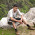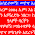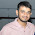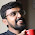## July 28, 2014

### Timing Analysis: Graph Based v/s Path Based

Hello folks! In this post, I'm gonna talk about the difference between two commonly used Static Timing Analysis methodologies, namely- the Graph Based Analysis and the Path Based Analysis.

I shall explain the difference with help of an example, shown below:
Now, we have two slews- fast and slow. In Graph Based Analysis, the worst slew propagation is ON, and the timing engine computes the worst case delays of all standard cells assuming the worst case slew for all the inputs of a gate. For example, while doing setup analysis in a graph-based methodology:
• The delay of the A-> Z (output) arc of the OR gate (in brown) would be computed assuming the worst slew, i.e. slew at pin B.
• Similarly, the delay of NAND gate (in blue) would be computed assuming the worst-case slew, i.e. the slew at pin A for both A-> Z arc as well as B-> Z arc.
• The delay of XOR gate (in yellow) would be computed assuming the worst slew, which happens to be the same at both A and B inputs.
• Finally, the delay of inverted AND would be computed assuming the slew of B pin for both the arcs.
While performing hold analysis in a graph-based methodology, the situation reverses, the the delays of all cells would be computed assuming the best slews (fast slews) for all standard cells!

This method of timing analysis is faster because the engine has to simply report the delay values of each cell and it more pessimistic! For example, for the OR gate, the delay for A-> Z arc would be less, but since the tool would compute the value assuming the slew of pin B, the delay would be higher and this adds unnecessary pessimism in the timing slack calculations. The Path-based analysis comes to the rescue at some cost.

In Path-based analysis, the tools takes into account the actual slew for the arcs encountered while traversing any particular timing path. For example for the path shown above from FF1 to FF2, the arcs encountered are- A-> Z for OR gate; B-> Z for NAND gate; B-> Z for XOR gate and A-> Z for the inverted AND gate.

The tool would therefore consider the actual slews and this dispenses with the unnecessary pessimism!

Why not use PBA instead of GBA? Who's stopping us?
The answer is the run-time. Since, PBA needs to compute the delays of standard cells in cognizance with the particular timing path, it incurs a penalty on the run-time to compute the delays, as opposed to GBA where a single slew was being used to compute the delays. In a nutshell, PBA is more accurate at the cost of run-time.

Typically, design engineers tend to use GBA for majority of the analysis. However, for the paths with a small violation (maybe of the order of 10s of ps) may be waived off by running PBA for the top-critical paths when the tape-out of the design is impending. One might argue that the extra effort spent in optimizing many other paths might have been saved had we used PBA earlier. And it is true! But like any engineering problem, there exists a trade-off and one needs to take a call between fixing the timing and a potential risk of delaying the tape-out!

#### 14 comments:

1.Suggest me a best institute for VLSI course

1.RV-VLSI bangalore

2.This comment has been removed by the author.

2.Best engineers I know learned while on the job.

3.Thanks a lot.

1.This comment has been removed by the author.

2.You're welcome, 朱古力男爵! :)

4.This comment has been removed by the author.

5.1.Thanks, Sarvang! :)

6.Very Helpful post.. nicely explained.
Thanks Guptaji.

-Harshad

7.Will delay ( delay_max_rise /delay_max_fall ....) in the above cases will be same or differnet ? If differnt then will tool be giving the exact values?

1.That's a good question considering the fact that I've shown transitions of only one polarity in the figure above. Now that I think about it, the STA tool (ETS or PT) would compute both the delays for a gate (delay_max_rise and delay_max_fall) while doing the setup analysis. In order to accomplish this, it will compute all slews (slew_max_rise, slew_max_fall) for all the inputs of the gate. While reporting the delay for let's say (positive unate gate) A -> Z, it will consider slew_max_rise at A to compute delay_max_rise of the gate. And slew_max_fall to compute delay_max_fall.

Similarly, while computing delay for arc B -> Z, it will do the same w.r.t the slews at B.

Optimization tools like EDI, ICC would follow Graph Based Analysis. So, they would consider the MAX(slew_max_rise @ A, slew_max_rise @ B) to compute delay_max_rise of the gate at Z for both A-Z and B-Z arcs. (Again assuming a positive unate gate). And similarly, would use MAX(slew_max_fall @ A, slew_max_fall @ B) to compute delay_max_fall for both A ->Z and B -> Z arc of the gate.

The above discussion might confound you more. Please let me know if there's something that's still not clear.

-Naman

8.Hi,
Can we do A PBA based analysis using the ETM libs ( as the internal flop to flop timing arc is not known ) will the tool perform PBA ?